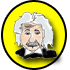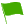Common Denominators (SHSAT Level):

Try your hand at some slightly more challenging common denominator problems similar to ones you are likely to see on exam day.

1.On a number line, points J, K, L, and M are in alphabetical order and point K lies at 3/8.

JK = 3 1/2

JM = 9 3/4

LM = 1 1/8

What is the position of point L?

Question 1 of 7

2.Jen’s class has between 30 and 41 students. Exactly 75% of the students in her class have red book bags, and exactly 1/6 of the students in her class do not have a book bag at all. How many students are in Jen’s class?

Question 2 of 7

3.A1A2A2A3A3A4A4A5A5A6

If Ak = 1/k for any positive value of k, and k is a positive integer, what is the value of the expression above?

Question 3 of 7

4.Calculate the multiplicative inverse of 4/3 + 1/2 - 4/5.

(express as a simple or improper fraction e.g. 2/3 or 3/2)

Question 4 of 7

5.a/4 - b/5 = ?

Question 5 of 7

6.5/6 + 2/5 = ?

Question 6 of 7

7.3/8 + 2/9 = ?

Question 7 of 7